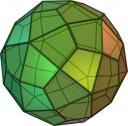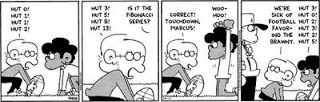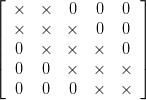# 50th Carnival of Mathematics

### Prologue

Tradition dictates that each Carnival of Mathematics begin with a riff about the number of the carnival. Since this is the 50th carnival, and the Roman numeral representation of 50 is L, I’ll start with a short riff on a few uses of “L”  in math.

• In tiling, “L” is for L-polyominos, like that look like the letter “L.”
• In analysis,  “L” is for Lp spaces, named after Henri Lebesgue. These are spaces of functions whose pth powers are Lebesgue-integrable.
• In statistics, “L” is for L-estimators. These are robust estimators formed by taking linear combinations of order statistics. I suppose in this case, “L” is for “linear.” The median is the simplest example of an L-estimator. Another simple L-estimator is John Tukey’s trimean.
• In number theory, “L” is for L-functions. First, there were Dirichlet’s L-functions. Then came, in order of increasing generality and abstraction, Hecke’s L-functions, Artin’s L-functions, and Weil’s L-functions. I don’t know where the “L” came from in this case. I suppose Dirichlet arbitrarily chose it and his successors followed his convention.

And now, on to recent math posts from around the web.

Ξ from 360 presents Hyperbolic Light, explaining why a lamp casts a hyperbolic pattern of light on a wall.Richard Elwes classifies polyhedra in Passing Platonic Solids. He starts with the most restrictive definition of regularity, which gives the five platonic solids. He then discusses which solids are possible as each of the criteria are removed.Fëanor from JOST A MON presents Puzzling Math in which he traces the history of the St. Ives problem, going back four thousand years.Continuing with the historical theme, Michael Croucher from Walking Randomly presents Full versions of historical mathematical texts (for free!).Cariel Montalban presents Basics of Linear Vector Spaces [Update: link went away] posted at Quantum Science Philippines, a blog for science students in the Philippines. Vector spaces are fundamental to quantum mechanics because wave functions reside in Hilbert spaces, vector spaces with inner products.

Rod Carvalho from Reasonable Deviations presents Matrix decompositions with sparsity constraints which asks a couple questions.

• Given a square matrix A and a particular sparsity pattern, how can we find if it is possible to factorize A into N square factor matrices (where N is finite) whose sparsity pattern is the specified one?
• If such factorization is possible, how can we compute each factor, and how can we find the value of N?Vlorbik from Vlorbik on Math Ed presents One Must Imagine Vlorbik Happy. His post looks at linear fractional transformations (Möbius transformations, except with real coefficients) in detail.

Finally, David Eppstein from 0xDE presents Why graphs?, an explanation of why graphs are so often used in computer science and software engineering.

### Announcements

The next Math Teachers at Play carnival for pre-K–12 math education will be March 6 at Let’s Play Math.

The next Carnival of Mathematics will be somewhere on March 13.

## 8 thoughts on “50th Carnival of Mathematics”

1. vlorbik

good job! here’s a gratuitous link
to the new “teachers at play” carnival.
long live the endeavour!

2. In the Foxtrot cartoon (about the Fibonacci numbers)… what is the pattern of numbers on the last panel?

3, 0, 2, 3, 2, 5

Is it OEIS A001608, Perrin sequence (or Ondrej Such sequence): a(n) = a(n-2) + a(n-3)?
http://www.research.att.com/~njas/sequences/A001608

Now why would the cartoon author think of that?

3. Angelina

Thanks for giving some space in the 50th Carnival of Maths to one of our posts. You have plenty of interesting articles here at The Endeavour. If not for Blog Carnival, we would not have stumbled upon your site. We will be your regular visitors!

4. Thank you – I’m honoured to be included! I love the hyperbolic light, and some other fascinating posts too.

5. Does anyone know where the next Carnival of Mathematics is supposed to be?

6. vlorbik

not me…

7. PiterKokoniz

Hi !!! ;)
My name is Piter Kokoniz. Just want to tell, that your blog is really cool